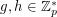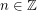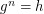# discrete log

## Discrete Logarithm Problem ★★★

Author(s):

Ifis prime and, we writeifsatisfies. The problem of finding such an integerfor a given(with) is the Discrete Log Problem.

Conjecture   There does not exist a polynomial time algorithm to solve the Discrete Log Problem.

Keywords: discrete log; NP